## Math 501: Differential Geometry

Homework 2 <me@tylerlogic.com>

February 1, 2013
http://coursework.tylerlogic.com/courses/math501/homework02

1 do Carmo pg 47 exercise 3

Curvature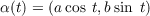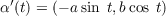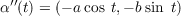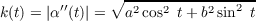Vertices Using k, we can determine the vertices of α by finding the values of t which make k(t) zero. Since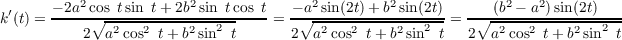then k(t) can only be zero when sin(2t) is zero, i.e. at t =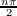for n . Since the domain is t [0,2π], then there are vertices at 0,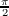, π,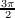, and 2π, however, α(0) = α(2π) so there are only four unqiue vertices at α(0) = (a,0), α(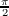) = (0,b), α(π) = (-a,0), and α(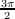) = (0,-b).

2 do Carmo pg 47 exercise 4

1 Denote the curve in question by α. The Fundamental Theory of The Local Theory of Curves allows us to translate and rotate the trace of α without affecting its curvature. So “move” the curve such that p is the origin and T align with the x-axis. Furthermore, reparametrize α by arc length, s, and such that α(0) = p and α(s) for positive s “heads towards” the line L.

With these changes, let’s evaluate the second order Taylor series exansion of α(s), given by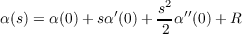(2.1)

where R is the sum of the higher order terms. We know that α(0) is p = (0,0). Because s parametrized α by arc length, then α(0) is the unit tangent vector at zero, which, since we aligned T with the x-axis, means simply that α(0) = (1,0). Furthermore, we know α′′(0) = kn to be perpendicular to α(0), meaning the normal vector n is (0,1), with the sign and magnitude of α′′(0) being determined by k. Substituting these realizations into Equation 2.1, we get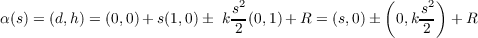where d and h are as defined in the problem’s statement. Thus denoting R by (Rx,Ry) we have that d = s + Rx and h = ± k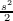+ Ry. The first equation can be rearranged as d-s = Rx, which tells us that s d as s 0 since Rx 0 as s 0. The second equation can be rearranged like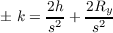and it informs us that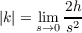since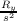0 as s 0, but because we saw above that s d as s 0, then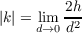3 do Carmo pg 47 exercise 5

2 Denote the curve contained inside of a circle by α. While maintaining the same center, shrink the circle, C, until it first touches α. Now either there are no points at which C and α differ or there is at least one. In the first case, this would mean α would trace out C in which case the curvature of α, |k|, would be 1∕r and certainly satisfies |k|≥ 1∕r. So assume that a point in α, call it q, differs from C. Denote by p, the point in the intersection of C and α such that |tq - tp| is minimal, where α(tq) = q and α(tp). There may be multiple such points p, but in that case, we can arbitrarily pick one and our argument still stands.

Now, making use of the Fundamental Theorem of The Local Theory of Curves, orient both α and C similarly to the previous problem, i.e. make p be the orgin and align the tangent vector of C at p with the x-axis. With this orientation, for a given d (defined as in the previous problem) hC hα. Because of this we have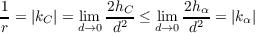by the previous problem, yielding |kα|≥ 1∕r as needed.

4 do Carmo pg 48 exercise 8 part a

Let α : [0,ℓ] 2 be a simple closed plane curve such that 0 < k c for some constant c. Because α has positive curvature that’s always less than c, then its curvature is always less than a circle of radius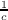. Since α is simple and closed, this implies that some circle of radius, call it S1, can be situated within and without intersection with the interior of the trace of α. Hence the area of the interior of α, A, is bounded below by the area of S1,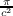. Thus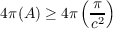and then making use of the Isoperimetric Inequality we get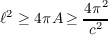or in other words,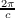, given the positivity of and c.

5

(a)

The mapping defined by t E(f + th) has a critical point at t = 0 because f minimizes E() and E(f + th) E(f) as t 0.

(b)

(c)

### References

   Rudin, Walter. Principles of Mathematical Analysis, 3rd ed. McGraw-Hill Inc. New York, 1976.